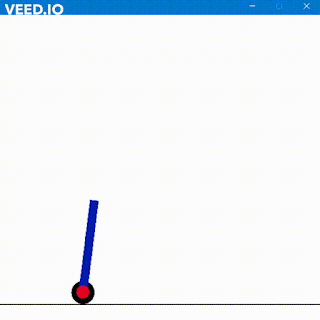### Frequency Divider by 4

Continued from here

To further divide the frequency we can use the output of D FF to the clock of another D FF and cascading the same we can divide the frequency by powers of 2.

The frequency can be f/2, f/4, f/8, f/16, ..... where f is frequency of clock.

For every division of frequency we need to add the code within circle. Here "a" is cascaded with "b" thus frequency output by "b" is f/4 and that of "a" is f/2. Further increase by n will output the frequency f/2n

Here is the block diagram for further understanding.

Here is the output

"q" is output from "b" with frequency f/4.
The "qbar" shown is of "a" with frequency of f/2.
Clock frequency is of f.

So Long